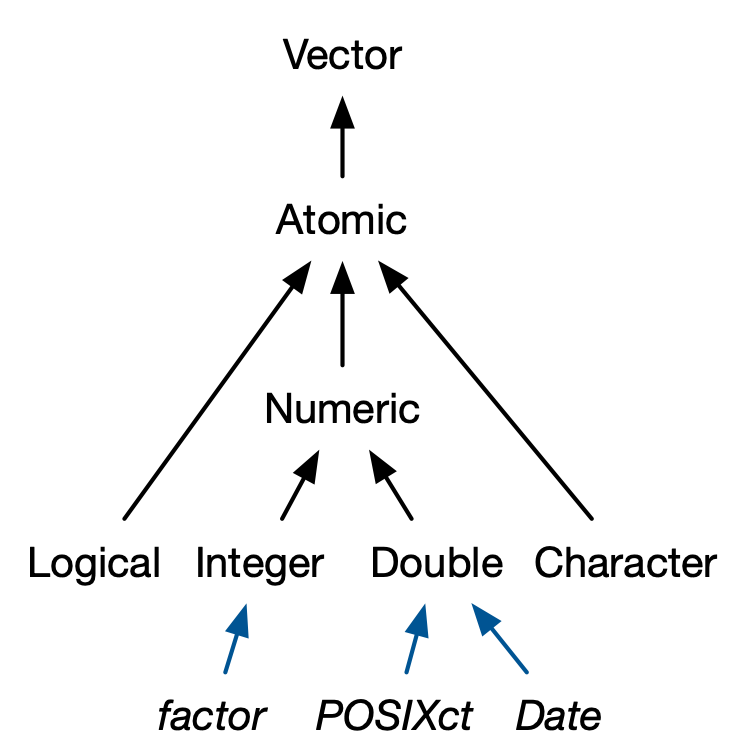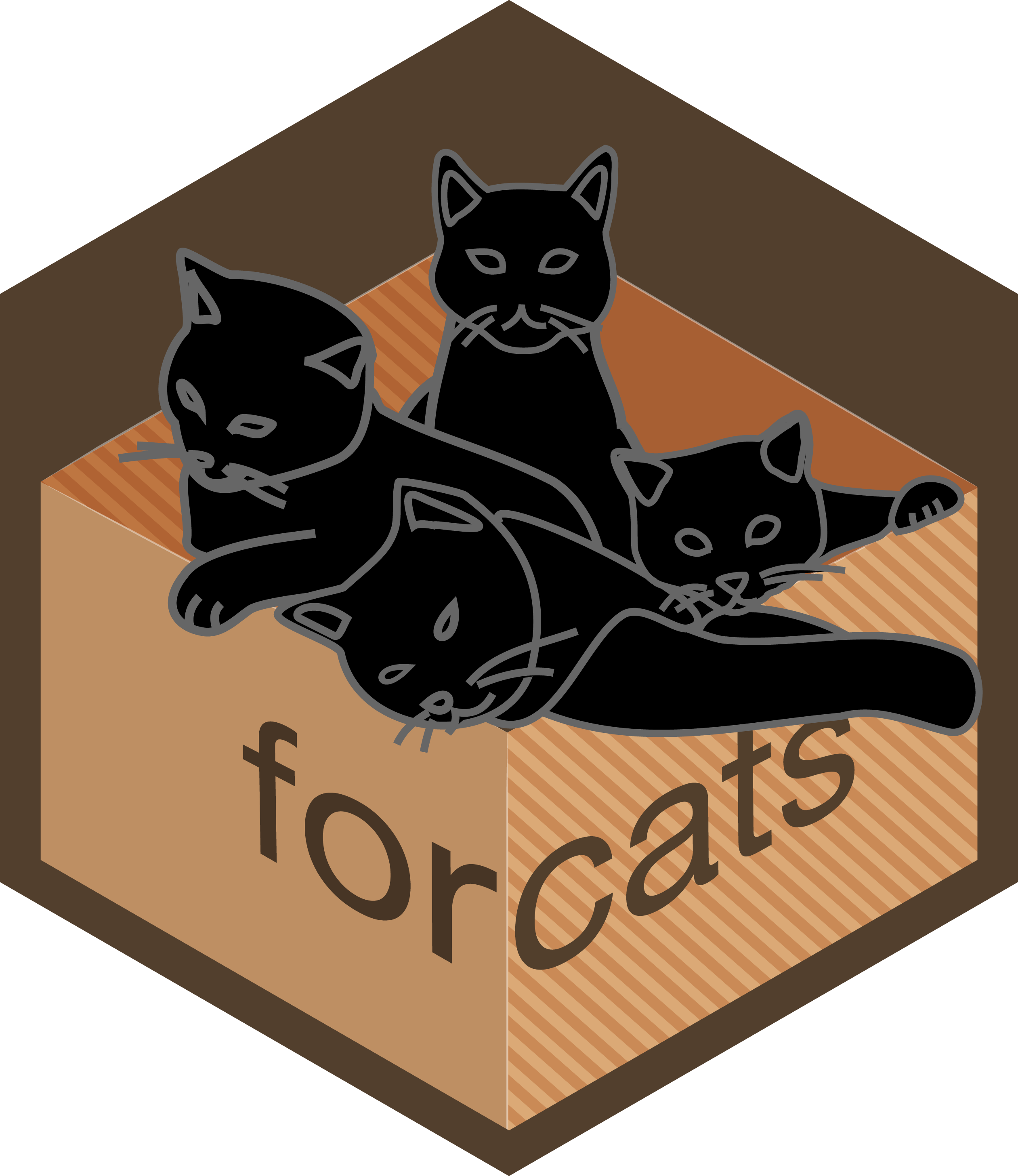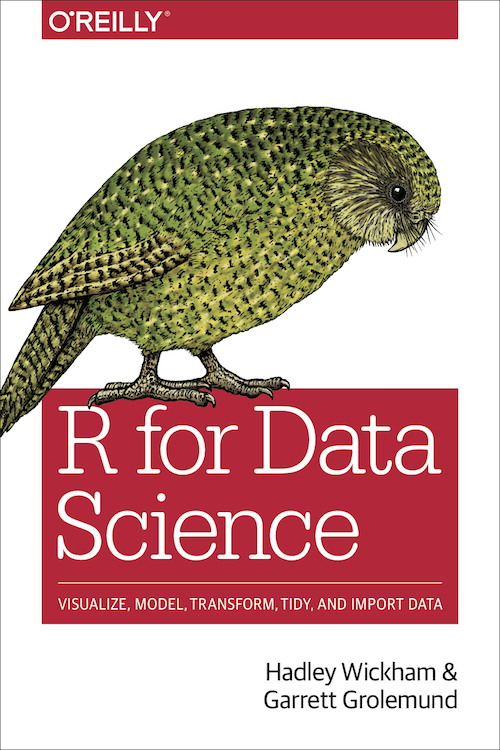--- title: "STATS 220" subtitle: "`r emo::ji('rocket')`Factors & date-times`r emo::ji('calendar')`" type: "lecture" date: "" output: xaringan::moon_reader: css: ["assets/remark.css"] lib_dir: libs nature: ratio: 16:9 highlightStyle: github highlightLines: true countIncrementalSlides: false --- class: middle center inverse ```{r initial, echo = FALSE, cache = FALSE, results = 'hide'} library(knitr) options(htmltools.dir.version = FALSE, htmltools.preserve.raw = FALSE, tibble.width = 60, tibble.print_min = 6) opts_chunk\$set( echo = TRUE, warning = FALSE, message = FALSE, comment = "#>", fig.path = 'figure/', cache.path = 'cache/', cache = FALSE, fig.retina = 3, fig.align = 'center', fig.width = 4.5, fig.height = 4, fig.show = 'hold', dpi = 120 ) ``` ```{r xaringan-panelset, echo = FALSE} xaringanExtra::use_panelset() ``` ```{r external, include = FALSE, cache = FALSE} read_chunk('R/05-fcts-dates.R') ```??? * forcats: my fav logo * lubridate: my fav pkg name --- ## Atomic vectors .pull-left[

.center[] ] .pull-right[ .center[] ] --- ```{r opts0, echo = FALSE} op <- options(width = 60) ``` .left-column[ .center[[](http://forcats.tidyverse.org)] ] .right-column[ ## Coerce to factors from one type ```{r dept-chr} ``` ```{r dept-fct, highlight.output = 3} ``` ] ```{r opts-exit0, echo = FALSE} on.exit(options(op), add = TRUE) ``` ??? * diff displays * fct: w meta info --- ```{r opts, echo = FALSE} op <- options(width = 40) ``` .pull-left[ ```{r chr} ``` ] .pull-right[ ```{r fct} ``` ] ```{r opts-exit, echo = FALSE} on.exit(options(op), add = TRUE) ``` --- ## Factors * Factors are used to represent a **categorical variable** in R. * There is a fixed and known set of possible values. * The fixed set of values is called the **levels** of the factor. .small[ ```{r fct-lvls} ``` ] --- ## Create factors * change the base level for modelling * display characters in a non-alphabetical order ```{r fct-create, highlight.output = 2} ``` ??? * data values not changing, only meta info levels changed --- ## Reorder factor levels to easily perceive patterns ## .center[`sci_tbl` ] .pull-left[ ```{r fct-vis, echo = FALSE, fig.width = 3.5, fig.height = 3.5} ``` ] .pull-right[ ```{r fct-ror, echo = FALSE, fig.width = 3.5, fig.height = 3.5} ``` ] ??? * in default order, takes time to process info --- ## Reorder factor levels to easily perceive patterns ## .center[`movies` ] .pull-left[ ```{r box-movies, echo = FALSE, fig.height = 3.5} ``` ] .pull-right[ ```{r box-movies-med, echo = FALSE, fig.height = 3.5} ``` ] --- ## `fct_reorder()` by sorting along another variable .pull-left[ .small[ ```{r fct-ror-p2, ref.label = "fct-ror", fig.width = 3.5, fig.height = 3.5} ``` ] ] .pull-right[ .small[ ```{r fct-ror2, fig.width = 3.5, fig.height = 3.5} ``` ] ] --- ## `fct_reorder()` by sorting along another variable ```{r fct-vct, highlight.output = 2} ``` --- ## `fct_reorder()` by sorting along another variable with `fun()` .pull-left[
```{r box-movies-med2, ref.label = "box-movies-med", eval = FALSE} ``` ] .pull-right[ ```{r box-movies-med3, ref.label = "box-movies-med", echo = FALSE, fig.height = 4.5} ``` ] --- ## `fct_infreq()` by counting obs with each level (largest first) .pull-left[

```{r col-movies, eval = FALSE} ``` ] .pull-right[ ```{r ref.label = "col-movies", echo = FALSE, fig.height = 4.5} ``` ] --- ## `fct_lump()` by lumping together factor levels into "other" .pull-left[

```{r col-movies-lump, eval = FALSE} ``` ] .pull-right[ ```{r ref.label = "col-movies-lump", echo = FALSE, fig.height = 4.5} ``` ] --- ## Convert numerics to factors: UoA grade scales .pull-left[ ```{r scores-sim} ``` ] .pull-right[ ```{r hist, fig.height = 3.5} ``` ] --- ## `cut()` numerics to factors ```{r cut} ``` ??? * underappreciated `cut()`, built-in function * include `0`, but doesn't matter for this data --- ## `fct_recode()` changes factor levels by hand ```{r recode} ``` ??? * Here I show the `fct_recode()`, manual work * what's other way to do this quickly --- .pull-left[ ```{r bar-range} ``` ] .pull-right[ ```{r bar-grade} ``` ] --- class: middle ## Your turn > What function can we use to replace `fct_recode()` for the `scores_tbl` data? `r countdown::countdown(minutes = 0, seconds = 30, font_size = "48px", top = 0)` ??? live demo: `fct_rev()` --- .left-column[ .center[[](http://lubridate.tidyverse.org)] ] .right-column[ `r emo::ji("arrow_down")` {lubridate} is NOT part of the core {tidyverse}, so load with ```{r lubridate} ``` Relative and exact time units: 1. An **instant** is a specific moment in time, such as January 1st, 2012. 2. An **interval** is a period of time that occurs between two specific instants. 3. A **duration** records the time span in seconds, it will have an exact length since seconds always have the same length. 4. A **period** records a time span in units larger than seconds, such as years, months, weeks, days, hours, and minutes. ] ??? * temporal data (recorded over time) is everywhere. Ur phone tracks your daily life. * instant: timestamp * leap seconds/years, time zones, DST * lab04, tz --- .pull-left[ ## `r emo::ji('calendar')`Dates ```{r dt} ``` ] .pull-right[ ## `r emo::ji('watch')`Date-times ```{r dttm} ``` ] --- ## Create date-times ```{r create-dttm} ``` --- ## Available time zones (~ 600`r emo::ji("bangbang")`) ```{r tz-name} ``` --- ## Parse date-times ```{r parse-dttm} ``` .pull-left[ * `ymd()`, `ymd_h()`, `ymd_hm()`, `ymd_hms()` * `dmy()`, `dmy_h()`, `dmy_hm()`, `dmy_hms()` ] .pull-right[ * `mdy()`, `mdy_h()`, `mdy_hm()`, `mdy_hms()` ] --- ## Extract components of date-times .pull-left[ ```{r extract} ``` ] .pull-right[ ```{r extract2} ``` ] --- ## Extract months/weekdays of date-times ```{r opts3, echo = FALSE} op <- options(width = 40) ``` .pull-left[ * month ```{r extract-mth} ``` ] .pull-right[ * weekday ```{r extract-wday} ``` ] ```{r opts3-exit, echo = FALSE} on.exit(options(op), add = TRUE) ``` --- ## Round, floor and ceiling date-times ```{r cal} ``` --- ## Perform accurate math on date-times ```{r opts4, echo = FALSE} op <- options(width = 40) ``` .small[ .pull-left[ ```{r arith} ``` ```{r arith1, highlight.output = 1} ``` ] .pull-right[ ```{r arith2} ``` ```{r arith3, highlight.output = 1} ``` ```{r arith4} ``` ] ] ```{r opts4-exit, echo = FALSE} on.exit(options(op), add = TRUE) ``` --- ## Format date-times (also coerce to characters) .pull-left[ .small[ ```{r fmt} ``` ] ] .pull-right[ * `a`/`A`: Abbreviated/full weekday name. * `b`/`B`: Abbreviated or full month name. * `m`: Month as decimal number (01-12 or 1-12). * `d`: Day of the month as decimal number (01-31 or 0-31) * `w`: Weekday as decimal number (0-6, Sunday is 0). * `y`/`Y`: Year without/with century. * more on [`?parse_date_time()`](https://lubridate.tidyverse.org/reference/parse_date_time.html) ] --- class: middle .pull-left[ ## `r emo::ji("film_projector")``movies` .small[ ```{r release-date-chr} ``` ```{r release-date, eval = FALSE} ``` ] ] .pull-right[ ```{r ref.label = "release-date", echo = FALSE, fig.height = 3.8} ``` ] --- ## Reading .pull-left[ .center[[](https://r4ds.had.co.nz)] ] .pull-right[ * [Factors](https://r4ds.had.co.nz/factors.html) * [Dates and times](https://r4ds.had.co.nz/dates-and-times.html) * [{forcats} cheatsheet](https://github.com/rstudio/cheatsheets/raw/master/factors.pdf) * [{lubridate} cheatsheet](https://rawgit.com/rstudio/cheatsheets/master/lubridate.pdf) ]